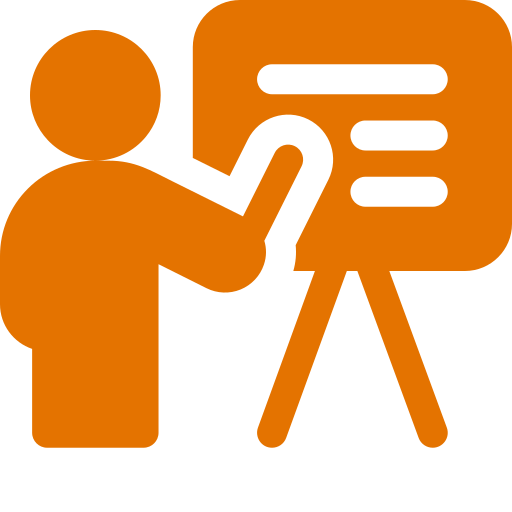# Problem Set #1: Introductory Exercises

### Objective

During this activity, students should be able to:

• Write some simple functions using the Clojure programming language.

This activity helps students develop the following skills, values and attitudes: ability to analyze and synthesize, capacity for identifying and solving problems, and efficient use of computer systems.

## Activity Description

Solve with your team the Clojure exercises described in this problem set. Make sure each function passes all the unit tests.

Create a namespace called introductory. At the begining of the file add the following code in order to declare the namespace and import the required external functions:

(ns introductory
(:require [clojure.test :refer [deftest is run-tests]])
(:require [clojure.math.numeric-tower :refer [sqrt]]))

(run-tests)

All the code you write should go between these two instructions.

1. Write a function called gibibytes->bytes that takes a number of $$\textit{gibibytes}$$ and returns its corresponding conversion to bytes.

Remember that:

• 1 KiB (kibibyte) = $$2^{10}$$ bytes = 1,024 bytes
• 1 MiB (mebibyte) = $$2^{20}$$ bytes = 1,024 kibibytes
• 1 GiB (gibibyte) = $$2^{30}$$ bytes = 1,024 mebibytes

Unit tests:

(deftest test-gibibytes->bytes
(is (= 0 (gibibytes->bytes 0)))
(is (= 1073741824 (gibibytes->bytes 1)))
(is (= 5368709120 (gibibytes->bytes 5)))
(is (= 26415122612224 (gibibytes->bytes 24601))))
2. Write a function called fahrenheit->celsius that takes a temperature in degrees Fahrenheit and converts it to degrees Celsius. Unit tests:

(deftest test-fahrenheit->celsius
(is (= 100.0 (fahrenheit->celsius 212.0)))
(is (= 0.0 (fahrenheit->celsius 32.0)))
(is (= -40.0 (fahrenheit->celsius -40.0))))

TIP: If $$F$$ is a temperature in degrees Fahrenheit, to convert it to $$C$$ degrees Celsius we must use the following formula:

$$C = \frac{5 (F - 32)}{9}$$
3. Write a function called sign that takes an integer value $$n$$. It returns -1 if $$n$$ is negative, 1 if $$n$$ is positive greater than zero, or 0 if n is zero. Unit tests:

(deftest test-sign
(is (= -1 (sign -5)))
(is (= 1 (sign 10)))
(is (= 0 (sign 0))))

4. Write a function called roots that returns a vector containing the two possible roots that solve a quadratic equation given its three coefficients ($$a$$, $$b$$, $$c$$) using the following formula:

$$x=\frac{-b\pm \sqrt{b^2-4ac}}{2a}$$

Unit tests:

(deftest test-roots
(is (= [-1 -1] (roots 2 4 2)))
(is (= [0 0] (roots 1 0 0)))
(is (= [-1/4 -1] (roots 4 5 1))))


TIP: To obtain the square root of a number, use the already imported sqrt function defined in the clojure.math.numeric-tower namespace.

5. The BMI (body mass index) is used to determine if a person's weight and height proportion is adequate. The BMI may be calculated using the following formula:

$$\textit{BMI} = \frac{\textit{weight}}{\textit{height}^2}$$

Where $$\textit{weight}$$ should be given in kilograms and $$\textit{height}$$ in meters. The following table shows how different BMI ranges are classified:

BMI range Description
BMI < 20 underweight
20 ≤ BMI < 25 normal
25 ≤ BMI < 30 obese1
30 ≤ BMI < 40 obese2
40 ≤ BMI obese3

Write a function called bmi that takes two arguments: weight and height. It should return a symbol that represents the corresponding BMI description computed from its input.

Unit tests:

(deftest test-bmi
(is (= 'underweight (bmi 45 1.7)))
(is (= 'normal (bmi 55 1.5)))
(is (= 'obese1 (bmi 76 1.7)))
(is (= 'obese2 (bmi 81 1.6)))
(is (= 'obese3 (bmi 120 1.6))))

6. Write a function called type-of-triangle that takes as input three numeric values that represent the sides of a triangle and returns a symbol indicating if the triangle is is equilateral, isosceles, or scalene.

#### Properties of a triangle

• A triangle is said to be equilateral if all its sides are equal.
• A triangle is said to be isosceles if exactly two of its sides are equal.
• A triangle is said to be scalene if none of its sides are equal.

Unit tests:

(deftest test-type-of-triangle
(is (= 'equilateral (type-of-triangle 3 3 3)))
(is (= 'equilateral (type-of-triangle 4.2 4.2 4.2)))
(is (= 'isosceles (type-of-triangle 4 4 3)))
(is (= 'isosceles (type-of-triangle 4 3 4)))
(is (= 'isosceles (type-of-triangle 3 4 4)))
(is (= 'scalene (type-of-triangle 1 2 3)))
(is (= 'scalene (type-of-triangle 7.1 6.4 9.2))))


## Deliverables

The program source file must include at the top the authors’ personal information (student ID and name) within comments. For example:

;----------------------------------------------------------
; Problem Set #1: Introductory Exercises
; Date: February 24, 2023.
; Authors:
;          A01770771 Sylvie Laufeydottir
;          A01777771 Loki Laufeyson
;----------------------------------------------------------


Also, each function should include a documentation string (docstring) with a brief description of its behavior. For example:

(defn max2
"Returns the largest of the two numbers x and y."
[x y]
(if (> x y) x y))


### Instrucciones para subir archivo

Para entregar el archivo introductory.clj, ingresa los siguientes datos:

Only one team member needs to upload the file.

Due date is Friday, February 24.

## Evaluation

This activity will be evaluated using the following criteria:

 -10 The program doesn't contain within comments the author’s personal information. A docstring is missing in one or more functions. The program contains syntax errors. The program was plagiarized in whole or in part. Depending on the amount of exercises that were solved correctly.